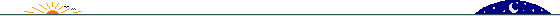Let the index of the Champernowne-continued-fraction terms be [0; 1, 2, 3, ...]. Here is a list of {index, number of digits in term} where the number of digits in the term exceeds 3:

{4, 6}

{18, 166}

{40, 2504}

{101, 140}

{162, 33102}

{246, 109}

{357, 2468}

{459, 136}

{526, 411100}

{638, 90}

{820, 2423}

{1051, 63}

{1221, 33056}

{1362, 95}

{1515, 2458}

{1627, 120}

{1708, 4911098} -> W

{1850, 69}

{2074, 2411}

{2175, 4}

{2309, 52}

{2364, 4}

{2528, 33005}

{2798, 12}

{3071, 2374}

{3090, 4}

{3160, 4}

{3339, 38}

{3569, 411044}

{3653, 5}

{3726, 84}

{3916, 2419}

{4141, 57}

{4311, 33051}

{4464, 97}

{4615, 2457}

{4745, 115}

{4838, 57111096} -> X

{5002, 49}

{5268, 2391}

{5545, 31}

{5810, 32985}

{6229, 4}

{6417, 2354}

{6729, 18}

{6871, 4}

{6992, 410979}

{7718, 2308}

{8065, 4}

{8469, 32939}

{8777, 4}

{9207, 2345}

{9827, 4911032} -> Y

{10034, 56}

{10279, 4}

{10292, 2393}

{10559, 29}

{10822, 32996}

{11439, 2359}

{11771, 26}

{11781, 4}

{12015, 411036}

{12202, 72}

{12345, 4}

{12414, 2405}

{12659, 45}

{12869, 33041}

{13054, 75}

{13251, 2438}

{13417, 101}

{13522, ?*} -> Z

The blue entries above represent the incremental size-records, suggesting two sequences: Position of the incrementally largest term in continued fraction for Champernowne (= index+1) and the incrementally largest numbers themselves, of which only 3 terms are shown in Sloane.

* On 4 September 2008, Mark Sofroniou determined this to be 651111094.2 May 2000 (updated 30 March 2010) © Rarebit Dreams